Electron. J. Differential Equations, Vol. 2021 (2021), No. 66, pp. 1-20.

Multiplicity and asymptotic behavior of solutions to fractional (p,q)-Kirchhoff type problems with critical Sobolev-Hardy exponent Xiaolu Lin, Shenzhou Zheng

Abstract:
Let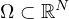be a bounded domain with smooth boundary and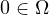. For 0<s<1, 1≤r<q<p, 0≤α<ps<N and a positive parameter λ, we consider the fractional (p,q)-Laplacian problems involving a critical Sobolev-Hardy exponent. This model comes from a nonlocal problem of Kirchhoff type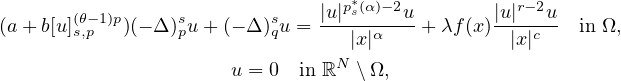where a,b>0, c<sr+N(1-r/p),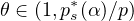and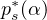is critical Sobolev-Hardy exponent. For a given suitable f(x), we prove that there are least two nontrivial solutions for small λ, by way of the mountain pass theorem and Ekeland's variational principle. Furthermore, we prove that these two solutions converge to two solutions of the limiting problem as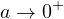. For the limiting problem, we show the existence of infinitely many solutions, and the sequence tends to zero when λ belongs to a suitable range.

Submitted January 12, 2021. Published August 10, 2021.
Math Subject Classifications: 35A15, 35B33, 35R11.
Key Words: Fractional (p,q)-Kirchhoff operators; critical Sobolev-Hardy exponent; multiple solutions; asymptotic behavior; symmetric mountain pass lemma.

Show me the PDF file (440 KB), TEX file for this article.Xiaolu Lin Department of Mathematics Beijing Jiaotong University Beijing 100044, China email: 19118003@bjtu.edu.cn Shenzhou Zheng Department of Mathematics Beijing Jiaotong University Beijing 100044, China email: shzhzheng@bjtu.edu.cn